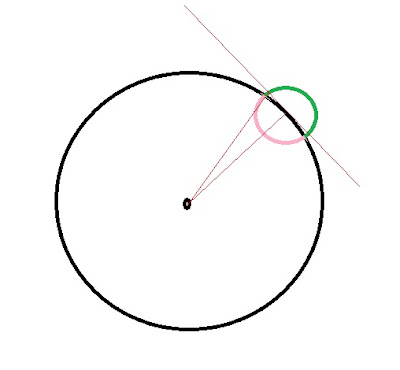## Monday, February 21, 2011

### The Drunkard's Walk

Yesterday I showed how you could take a piece of the energy spectrum and convert it into an actual waveform. You just approximate the energy distribution by a series of sine waves, pick an arbitrary bandwidth, and add up the waves. The picture you end up with depends on how finely you chop up the distribution into discrete waves, and how many of those waves you choose to add up.

In each case you get series of wave trains of a certain duration, amplitude, repetition rate. Some examples of what you might get from a given distribution are:

10 mV/m pulses 4 microsecond duration 100 kHz repetition rate
40 mV/m pulses 1 microsecond duration 100 kHz repetition rate
20 mV/m pulses 4 microsecond duration 25 kHz repetition rate

(These aren't the exact values I worked out yesterday, but they illustrate the general pattern: multiply column A by column B and the square root of column C to get a constant value.)

I then claimed that no matter what choices you make, you will get the exact same result when you apply the resulting waveform the the problem of finding the equilibrium of a mechanical resonator in that field. Now I'm going to show why it works. To understand the reason we must first consider a famous math problem known as the Drunkard's Walk.

The premise of the problem is that you have a drunk standing under a lamppost and he begins to wander aimlessly. Every second he takes one step, and each step is one meter long. The question is, how far from the lamppost will he be found after a given time?

It's not such an easy question, but the answer turns out to be simple: in this example it's just the square root of the number of steps. So after 100 seconds the drunk is expected to be found (on average) ten meters from the lamppost.

Why should this be? Since his steps are totally random, shouldn't he make absolutely no progress on average?

That's also true. His most likely progress in any given direction is zero, and is most likely endpoint is...right where he started. But his average distance away from the lamppost is still ten meters. Go figure.

It's actually not hard to see why he must, on average, continue to get farther and farther away. Suppose at some time he happens to be five meters away from the lamppost. His next step will place him somewhere on an imaginary circle exactly one meter from his present location.

If you look at that circle, you will see that most of it (more than half of it, at least) lies outside a five-meter radius from the lamp!If you look at the small circle you see that the segment of arc (in green) outside the five-meter radius is longer than the segment (in purple) inside five meters. This is true wherever he starts from; and so, on average, the drunk keeps getting farther from the lamppost.
How much farther? That's a tough one. But there is a little trick that will let us guess the answer. The most "neutral" thing the drunk can do is, arguably, to take a step perpendicular to the radius of the big circle. We can then use the Law of Pythagoras to see where he ends up. 5^2 plus 1^2 equals 26, so his distance from the lamppost is the square root of 26. Since his previous location at a distance of 5 represented the square root of 25, we see that this little trick gives us exactly the answer that we wanted...the distance goes as the square root of the number of steps.
We're going to need this trick when we go back to the harmonic oscillator.